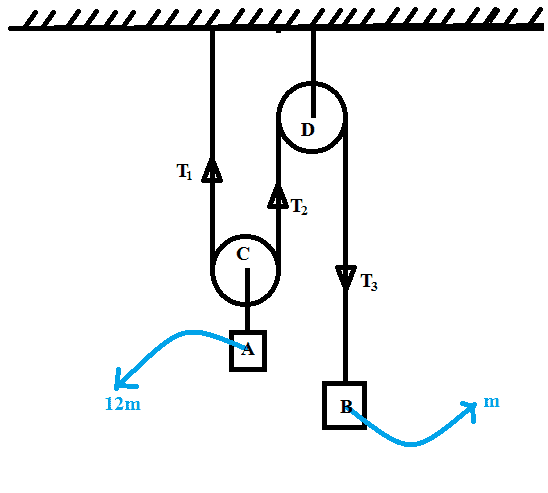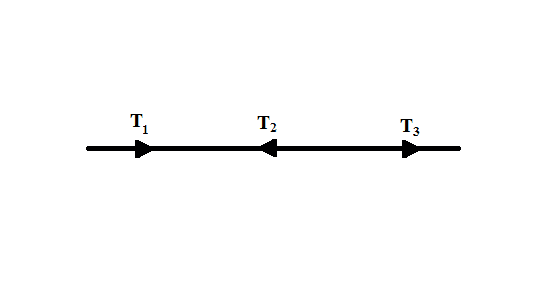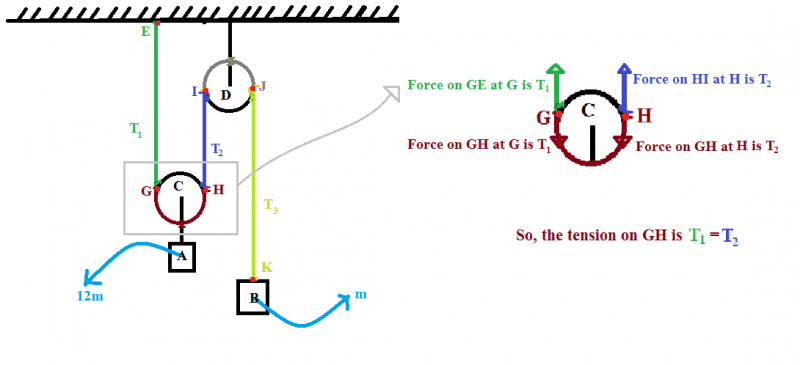# Tensions of cable over a pulley

[moderator's note: moved to homework forum so no template]This is a frictionless ideal pulley.
Are $T_1, T_2, T_3$ equal? If so, why? Please explain in details?

Last edited by a moderator:

Philip Wood
Gold Member

Probably, I have mistaken the direction of $T_1$ and $T_2$. They should be downward.
Then I straightened the cable.So, I assume, $T_1 + T_3 - T_2 = 0$ .But 'why', I am not sure.

Philip Wood
Gold Member
Right. Now we have something to go on.

Tensions aren't really forces, though they're closely related. Suppose A and B pull each other in a tug of war, with a rope which at a particular time has a tension of 200 N. That means that the rope pulls A with a force of 200 N, and pulls B with a force of 200 N. These forces are equal and opposite. So in your diagram in post 1, the arrows don't show tensions, but forces. Specifically the two left hand arrows show the forces that the rope exerts on the bottom pulley and the right hand arrow shows the force that the rope exerts on the top pulley. This could cause confusion. It's often best to draw separate diagrams for separate parts of the system showing the forces acting – so-called free body diagrams.

Now your question. The tension in the rope doesn't have a direction (in the same sense that a force has a direction). But it can move freely over the pulleys, and you are right to think that it can be regarded as straight from the point of view of comparing the tensions in the various sections of it. What do you conclude about these tensions? Note that the arrows you put on the straightened rope in post 3 should be removed, as we're dealing with tension, not force. Your equation is also wrong.

Right. Now we have something to go on.

Tensions aren't really forces, though they're closely related. Suppose A and B pull each other in a tug of war, with a rope which at a particular time has a tension of 200 N. That means that the rope pulls A with a force of 200 N, and pulls B with a force of 200 N. These forces are equal and opposite. So in your diagram in post 1, the arrows don't show tensions, but forces. Specifically the two left hand arrows show the forces that the rope exerts on the bottom pulley and the right hand arrow shows the force that the rope exerts on the top pulley. This could cause confusion. It's often best to draw separate diagrams for separate parts of the system showing the forces acting – so-called free body diagrams.

Now your question. The tension in the rope doesn't have a direction (in the same sense that a force has a direction). But it can move freely over the pulleys, and you are right to think that it can be regarded as straight from the point of view of comparing the tensions in the various sections of it. What do you conclude about these tensions? Note that the arrows you put on the straightened rope in post 3 should be removed, as we're dealing with tension, not force. Your equation is also wrong.So, now I think $T_1 = T_2 = T_3$. I explained the reason in the picture. Please check it whether I am right or Wrong.

Philip Wood
Gold Member
You are right. The tension is the same (call it T) throughout the rope if the pulleys are frictionless. The rope therefore exerts an upward force of 2T on the left hand pulley, a downward force of 2T on the right hand pulley (which is counteracted by an equal and opposite force in the rope holding it to the ceiling) and an upward force of T on mass B.

•arpon
haruspex